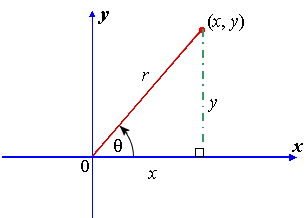Search IntMath
Close

450+ Math Lessons written by Math Professors and Teachers

5 Million+ Students Helped Each Year

1200+ Articles Written by Math Educators and Enthusiasts

Simplifying and Teaching Math for Over 23 Years

# 1. Fundamental Trigonometric Identities

by M. Bourne

Proving out fundamental trigonometric identities and diving into uses for solving problems.

After we revise the fundamental identities, we learn about:
Proving trigonometric identities

But before we start to prove trigonometric identities, let's see where the basic identities come from.

Recall the reciprocal trigonometric functions, csc θ, sec θ and cot θ from the trigonometric functions chapter:

csc theta=1/(sin theta)

sec theta=1/(cos theta)

cot theta=1/(tan theta)

Now, consider the following diagram where the point (x, y) defines an angle θ at the origin, and the distance from the origin to the point is r units:From the diagram, we can see that the ratios sin θ and cos θ are defined as:

sin theta=y/r

and

cos theta=x/r

Now, we use these results to find an important definition for tan θ:

(sin theta)/(cos theta)=(y/r)/(x/r)=y/rxxr/x=y/x

Now also tan theta=y/x, so we can conclude:

tan theta=(sin theta)/(cos theta)

## Ratios based on Pythagoras' Theorem

Also, for the values in the above diagram, we can use Pythagoras' Theorem and obtain:

y2 + x2 = r2

Dividing through by r2 gives us:

y^2/r^2+x^2/r^2=1

so we obtain the important result:

sin^2\ theta + cos^2\ theta = 1

## Related Formulas

We now proceed to derive two other related formulas that can be used when proving trigonometric identities.

It is suggested that you remember how to find the identities, rather than try to memorise each one.

Dividing sin2 θ + cos2 θ = 1 through by cos2 θ gives us:

(sin^2 theta)/(cos^2 theta)+1=1/(cos^2\ theta)

so

tan^2 theta + 1 = sec^2 theta

Dividing sin2 θ + cos2 θ = 1 through by sin2 θ gives us:

1+(cos^2 theta)/(sin^2 theta)=1/(sin^2 theta

so

1 + cot^2 theta = csc^2 theta

## Trigonometric Identities Summary

tan theta=(sin theta)/(cos theta)

sin^2 theta+cos^2 theta=1

tan^2 theta+1=sec^2 theta

1+cot^2 theta=csc^2 theta

## Proving Trigonometric Identities

Suggestions...

1. Learn well the formulas given above (or at least, know how to find them quickly). The better you know the basic identities, the easier it will be to recognise what is going on in the problems.
2. Work on the most complex side and simplify it so that it has the same form as the simplest side.
3. Don't assume the identity to prove the identity. This means don't work on both sides of the equals side and try to meet in the middle. Start on one side and make it look like the other side.
4. Many of these come out quite easily if you express everything on the most complex side in terms of sine and cosine only.
5. In most examples where you see power 2 (that is, 2), it will involve using the identity sin2 θ + cos2 θ = 1 (or one of the other 2 formulas that we derived above).

Using these suggestions, you can simplify and prove expressions involving trigonometric identities.

### Example 1

Prove that

(tan y)/(sin y)=sec y

We express everything in terms of sin y and cos y and then simplify.

("LHS" stands for "left-hand side" of the equation and "RHS" means "right-hand side".)

"LHS"=tan\ yxx1/(sin y)

=(sin y)/(cos y)xx1/(sin y)

=1/(cos y)

=sec\ y

="RHS"

### Example 2

Prove that

sin y + sin y\ cot^2y = csc y

"LHS" = sin y + sin y\ cot^2y

=sin y(1+cot^2y)

=sin y(csc^2y)

=sin y 1/(sin^2y)

=1/(sin y)

 = csc\ y

="RHS"

### Example 3

Prove that

sin x\ cos x\ tan x = 1 − cos^2x

"LHS" = sin x\ cos x\ tan\ x

=sin x\ cos x(sin x)/(cos x)

=sin^2x

=1-cos^2x   (since sin^2x+cos^2x=1)

="RHS"

### Exercises

1. Prove that

tan x + cot x = sec x\ csc x

"LHS"=tan\ x + cot\ x

=(sin x)/(cos x) + (cos x)/(sin x)

=(sin^2x+cos^2x)/(cos x\ sin x)

=1/(cos x\ sin x)

=1/(cos x)xx1/(sin x)

=sec\ x\ csc\ x

="RHS"

2. Prove that

(1+cos x)/(sin x)=(sin x)/(1-cos x)

In this problem, it is easier to start from the RHS. We need to perform a trick.

We multiply numerator and denominator of the fraction by the conjugate of the denominator. (We saw this idea in the sections Rationalizing the Denominator (in surds) and in dividing complex numbers.)

This will give us an expression in the denominator that we can simplify, using sin2 θ + cos2 θ = 1.

"RHS" = (sin x)/(1-cos x)

=(sin x)/(1 - cos x)xx (1 + cos x)/(1 + cos x)

=((sin x)(1 + cos x))/(1 - cos^2 x)

=((sin x)(1 + cos x))/(sin^2 x)

=(1 + cos x)/(sin x)

="LHS"

## Problem Solver

This tool combines the power of mathematical computation engine that excels at solving mathematical formulas with the power of GPT large language models to parse and generate natural language. This creates math problem solver thats more accurate than ChatGPT, more flexible than a calculator, and faster answers than a human tutor.